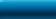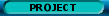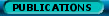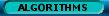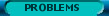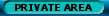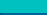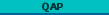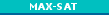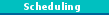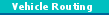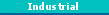edited by Thomas Stützle and Luis Paquete, INTELLEKTIK

## Contents

The quadratic assignment problem (QAP) is an important problem in theory and practice. It was introduced by Koopmans and Beckmann in 1957 and is a model for many practical problems like backboard wiring, campus and hospital layout, typewriter keyboard design, scheduling and many others can be formulated as QAPs. Intuitively, the QAP can best be described as the problem of assigning a set of facilities to a set of locations with given distances between the locations and given flows between the facilities. The goal then is to place the facilities on locations in such a way that the sum of the product between flows and distances is minimal.

More formally, given n facilities and n locations, two n . n matrices A = [a_ij] and B=[b_rs], where a_ij is the distance between locations i and j and b_rs is the flow between facilities r and s, the QAP can be stated as follows:

```
---  ---
\    \
min   /    /    a          b
p    ---  ---   p(i),p(j)  ij
i    j

```
where S(n) is the set of all permutations (corresponding to the assignments) of the set of integers {1,... ,n}, and p(i) gives the location of facility i in the current permutation p. Here b_ij a_[p(i) p(j)] describes the cost contribution of simultaneously assigning facility i to location p(i) and facility j to location p(j). The QAP is a NP-hard optimization problem. It is considered as one of the hardest optimization problems, because exact algorithms show a very poor performance on the QAP. In fact, the largest, non-trivial instance solved to optimality today is of size n=30! Therefore, to practically solve the QAP one has to apply heuristic algorithms. Several such heuristic algorithms have been proposed which include algorithms like iterative improvement, simulated annealing, tabu search, genetic algorithms, evolution strategies, GRASP, ant algorithms, scatter search, and iterated local search.

T. Stützle and M. Dorigo, 2001. Local Search and Metaheuristics for the Quadratic Assignment Problem. Technical Report AIDA-01-01, Intellectics Group, Darmstadt University of Technology, Germany.

R.E. Burkard, E. Cela, P.M. Pardalos and L. Pitsoulis. The quadratic assignment problem. In P.P. Pardalos and M.G.C. Resende, editors, Handbook of Combinatorial Optimization, 1998. Kluwer Academic Publishers, Dordrecht, pp. 241-238.

E. Cela. The Quadratic Assignment Problem: Theory and Algorithms. Kluwer Academic Publishers, Dordrecht, 1998.

P.M. Pardalos, F. Rendl, and H. Wolkowicz. The quadratic assignment problem: a survey of recent developments. In P. Pardalos and H. Wolkowicz, editors, Quadratic assignment and related problems, volume 16, pages 1-42. DIMACS Series in Discrete Mathematics and Theoretical Computer Science, 1994.Network Coordinator: Marco Dorigo    e-mail: mdorigo@ulb.ac.be    Web site responsibles: Christian Blum and Max Manfrin